# Data Analysis

## Ensembles.1

1. Some materials are taken from machine learning course of Victor Kitov

# Let's recall previous lecture¶

## Dimention reduction¶

• PCA - finds directions along which our datapoints have the greatest variance
• Can estimate importance of each component via correspondent eigenvalue

# Ensembles¶

## Loss vs model complexity¶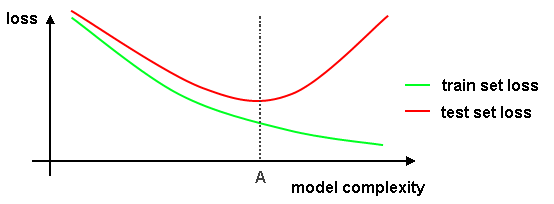• expected loss on test set is always higher than on train set

# Bias-variance decomposition¶

## Bias-variance decomposition¶

• Consider regression task and MSE
• Let $y$ - be target features, $x$ - are predictors
• True relationship: $y(x) = f(x) + \epsilon$ where $\epsilon$ is gaussian white noise, $\epsilon \sim \mathcal{N}(0,\sigma^2)$
• Model: $a(x) = \hat{y}$.
\begin{equation} \begin{split} Error & = \mathbb{E}_{X,Y,\epsilon}\left[(a(x) - y(x))^2\right] = \\ & = \mathbb{E}_{X,Y}\left[(a(x) - \mathbb{E}_{X,Y}[a(x)])^2\right] + \left(\mathbb{E}_{X,Y}\left[a(x)\right] - f(x)\right)^2 + \sigma^2 = \\ & = \text{Variance} + \text{Bias}^2 + \text{Noise} \end{split} \end{equation}

Those components can be interpreted as

• $\text{Variance}$ (Разброс) - model's sensivity to dataset. High variance usually means that model is overfitted.
• $\text{Bias}$ (Смещение) - is in charge of model's precision. High bias usually means that model is underfitted.
• $\text{Noise}$ (Шум) - that is just noise

## Bias and variance¶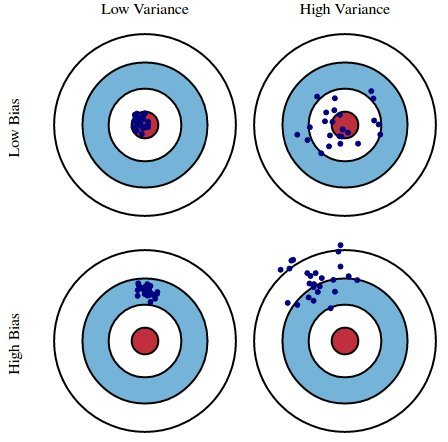## Bias-variance decomposition¶

Define for brevity of notation $y(x)=y,\, a(x) = a,\, f(x) = f, \, \mathbb{E}=\mathbb{E}_{X,Y,\varepsilon}$:

\begin{equation} \begin{split} \text{Error} & = \mathbb{E}\left[(a - y)^2\right] = \mathbb{E}\left[a^2 - 2ay + y^2\right] = \\ & = \underbrace{\mathbb{E}\left[(a - \mathbb{E}[a])^2\right] + \mathbb{E}[a]^2}_{=\mathbb{E}[a^2]} - 2\mathbb{E}[ay] + \underbrace{\mathbb{E}\left[(y - \mathbb{E}[y])^2\right] + \mathbb{E}[y]^2}_{=\mathbb{E}[y^2]} = \\ & = \mathbb{E}\left[(a - \mathbb{E}[a])^2\right] + \left(\mathbb{E}\left[a\right] - f\right)^2 + \mathbb{E}\left[(y - f)^2\right] = \\ & = \text{Variance} + \text{Bias}^2 + \text{Noise} \end{split} \end{equation}

## Ensemble learning¶

Ensemble learning - using multiple machine learning methods for a given problem and integrating their output to obtain final result

Synonyms: committee-based learning, multiple classifier systems.

Applications:

• supervised methods: regression, classification
• unsupervised methods: clustering

## Ensembles use cases¶

• underfitting, high model bias

• existing model hypothesis space is too narrow to explain the true one
• different oversimplified models have bias in different directions, mutually compensating each other.
• overfitting, high model variance

• avoid local optima of optimization methods
• too small dataset to figure out concretely the exact model hypothesis
• when task itself promotes usage of ensembles with features of different nature
• E.g. computer security:
• multiple sources of diverse information (password, face detection, fingerprint)
• different abstraction levels need to be united (current action, behavior pattern during day, week, month)

# Accuracy improvement demos¶

## Classification¶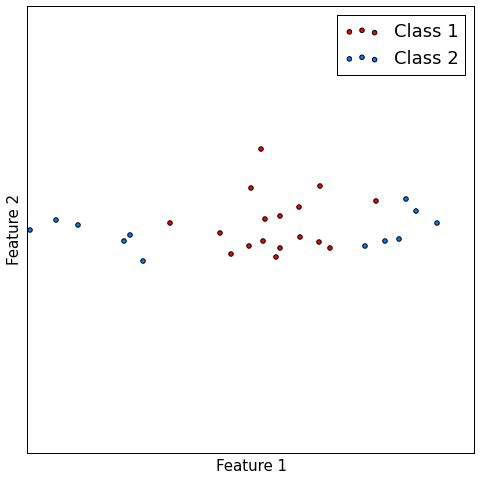## Classification¶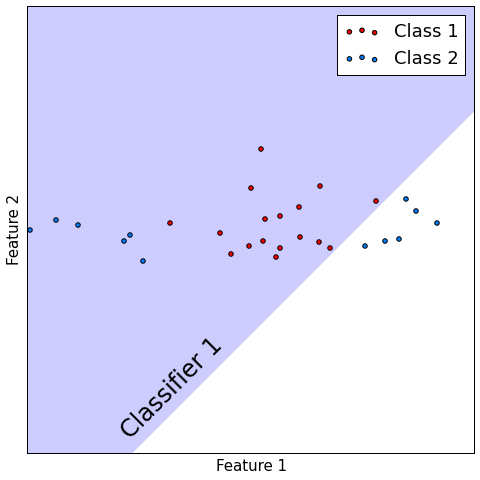## Classification¶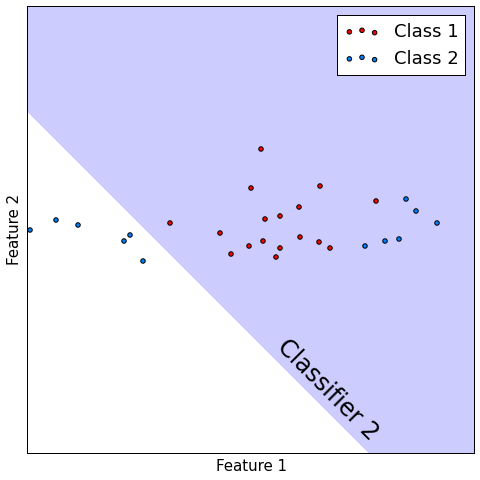## Classification¶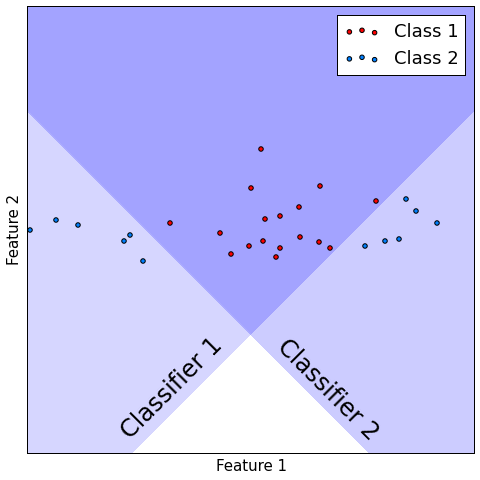## Classification¶

• Consider $M$ classifiers $f_{1}(x),...f_{M}(x)$, performing binary classification.
• Let probability of correct precision be constant $p\in(\frac{1}{2}, 1)$: $p(f_{m}(x)=y)=p\,\forall m$
• Suppose all models make mistakes or correct guesses independently of each other.
• Let $F(x)$ be majority voting combiner.
• Then $p(F(x) = y)\to1$ as $m\to\infty$
In :
M = 50
T = M//2 + 1
p = 0.6
p_ens = sum([scipy.misc.comb(M,k)*(p**k)*((1-p)**(M-k)) for k in range(T, M+1)])
p_ens

Out:
0.9021926358467505

## Regression¶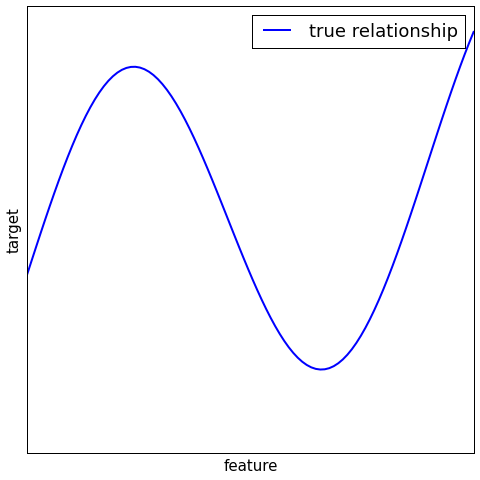## Regression¶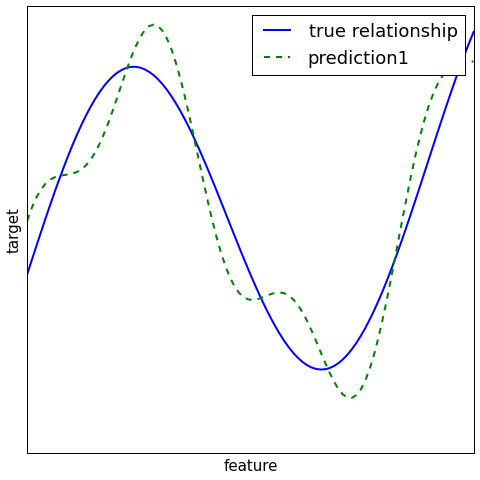## Regression¶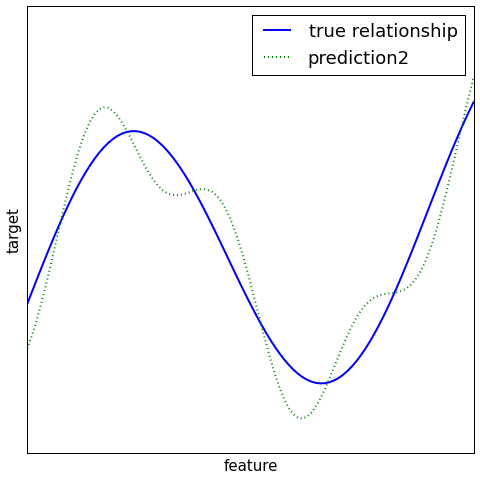## Regression¶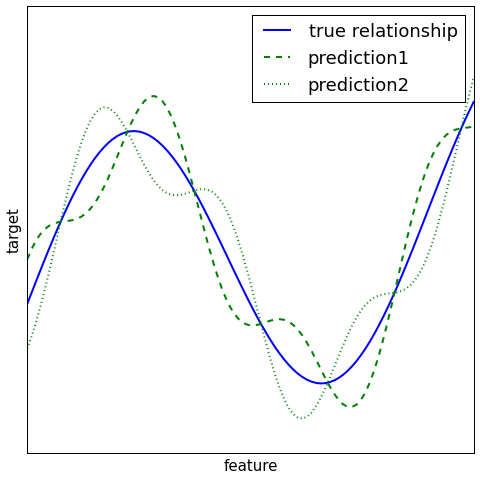# Fixed integration schemes for classification¶

## Fixed integration schemes for classification¶

Output of base learner k: exact class: $\omega_{1}$ or $\omega_{2}$.

Combiner predicts $\omega_{1}$ if:

• all classifiers predict $\omega_{1}$ (AND rule)
• at least one classifier predicts $\omega_{1}$ (OR rule)
• at least $k$ classifiers predict $\omega_{1}$ (k-out-of-N)
• majority of classifiers predict $\omega_{1}$ (majority vote)

Each classifier may be assigned a weight, based on its performance:

• weighted majority vote
• weighted k-out-of-N (based on score sum)

## Fixed combiner - ranking level¶

Output of base learner k:

Ranking of classes: $$\omega_{k_{1}}\succeq\omega_{k_{2}}\succeq\ldots\succeq\omega_{k_{C}}$$

Ranking is equivalent to scoring of each class (with incomparable scoring between classifiers).

Definition
Let $B_{k}(i)$ be the count of classes scored below $\omega_{i}$ by classifier $k$.

Borda count $B(i)$ of class $\omega_{i}$ is the total number of classes scored below $\omega_{i}$ by all classifiers: $$B(i)=\sum_{k=1}^{K}B_{k}(i)$$

Combiner predicts $\omega_{i}$ where $i=\arg\max_{i}B(i)$

## Fixed combiner at class probability level¶

Output of base learner k: vectors of class probabilities: $$[p^{k}(\omega_{1}),\,p^{k}(\omega_{2}),\,\ldots\,p^{k}(\omega_{C})]$$

Combiner predicts $\omega_{i}$ if $i=\arg\max_{i}F(p^{1}(\omega_{i}),p^{2}(\omega_{i}),\ldots p^{K}(\omega_{i}))$

• $F$ = mean or median.

# Sampling ensemble methods¶

## Sampling ensembles¶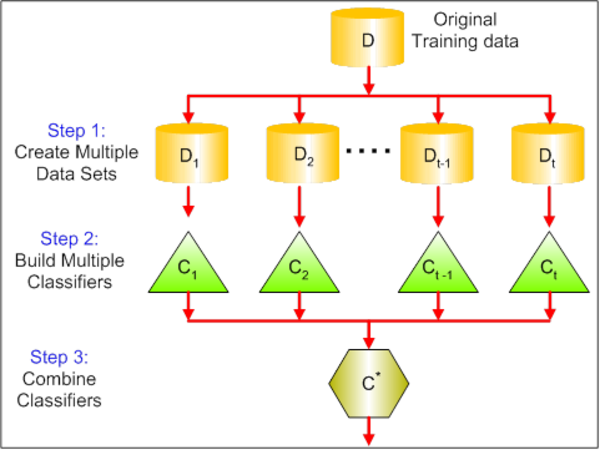## How bagging affects bias-variance decomposition?¶

Intuitively, bagging should reduce variance

• Consider $z_1,\dots,z_n~Z$, i.i.d., $\mathbb{E}[Z] = \mu$, $Var[Z] = \sigma^2$
• How can we estimate $\mu$?
• We can use any single $z_i$, but it would be quite uncertain, since $Var[z_i] = \sigma^2$
• What about $\frac{1}{n}\sum_i z_i$ ?
• $\mathbb{E}\left[\frac{1}{n}\sum\limits_i z_i\right] = \mu$, but!
• $Var\left[\frac{1}{n}\sum\limits_i z_i\right] = \frac{1}{n^2}Var\left[\sum\limits_i z_i\right] = \frac{1}{n}\sigma^2$
• Variance of that estimation is lower
• Say we have $n$ independent training sets from the same distribution
• Learning $n$ alorithms on them would provide us with $n$ decision functions $a_i(x)$
• Average prediction of an object $x$ is $$a_{avg}(x) = \frac{1}{n}\sum_{i=1}^n a_i(x)$$
• $\mathbb{E}[a_{avg}(x)] = \mathbb{E}[a_1(x)]$
• $Var[a_{avg}(x)] = \frac{1}{n}Var[a_1(x)]$
• ...
• Profit (?)

## Bagging¶

• Need a way to make independent training sets (independent decision functions)
• One of the ways is bootstrap:
• Generate $B$ datasets from original $X$ using sampling with replacements - $X_i$
• Each generated dataset contains about $67\%$ of original dataset
• Train a model $a_i(x)$ on each dataset
• Average their preditions
$$a_{bag} = \frac{1}{B}\sum_{i=1}^B a_i(x)$$## Bagging & random subspaces¶

### Bagging¶

• random selection of samples (with replacement)
• efficient for methods with high variance w.r.t. $X,Y$.

### Random subspace method¶

• random selection of features (without replacement)

# Random forests¶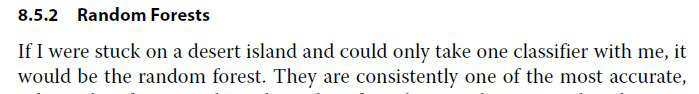# Random forests¶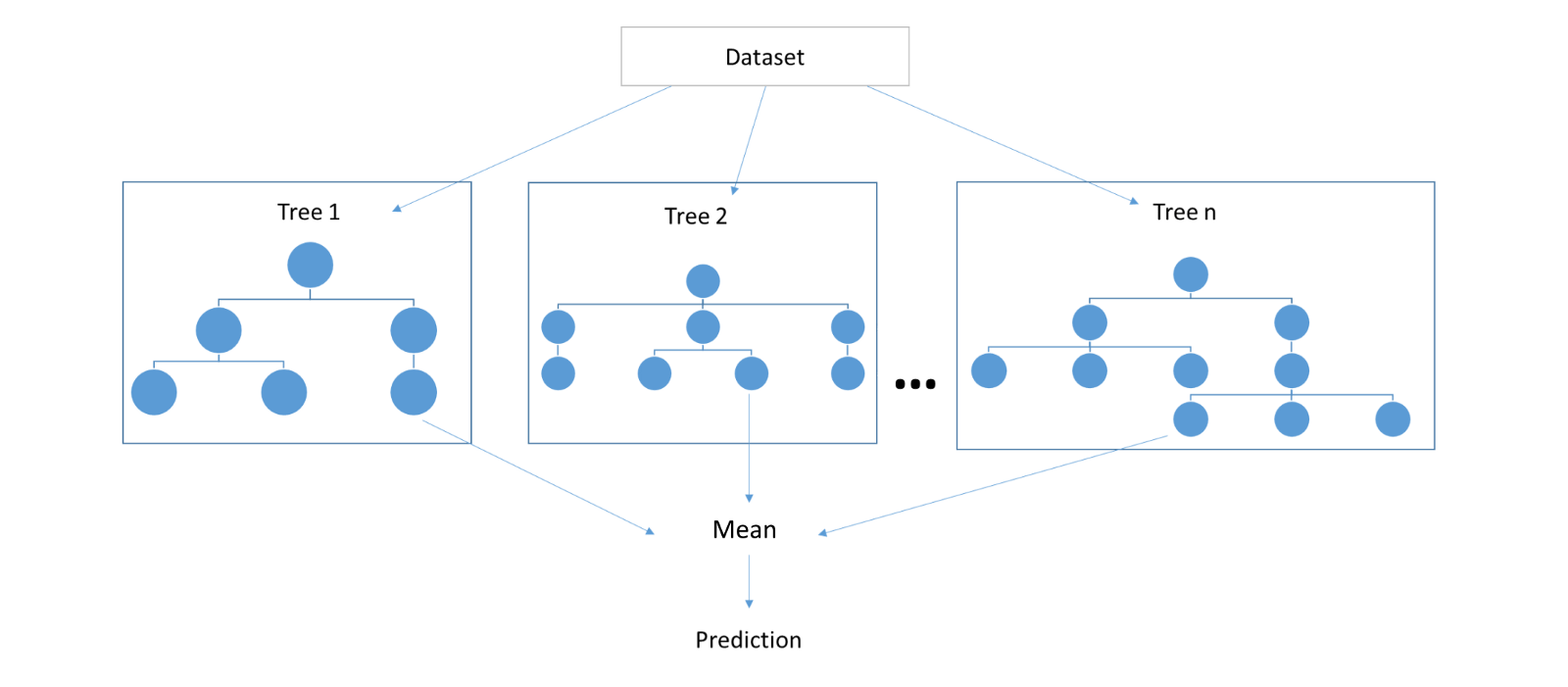## Random forests¶

• In fact, bootstraped datasets are not independent w.r.t. $p(x,y)$
• Random forests tries to make trees even more independent through randomization in construction algorithm

Input:

• training dataset $TDS=\{(x_{i},y_{i}),\,1=1,2,...N\}$;
• the number of trees $B$ and the size of feature subsets $m$.

for $b=1,2,...B$:

1. generate random training dataset $TDS^{b}$ of size $N$ by sampling $(x_{i},y_{i})$ pairs from $TDS$ with replacement (bootstrap)
2. build a tree using $TDS^{b}$ training dataset with feature selection for each node from random subset of features of size $m$ (generated individually for each node).

Output: $B$ trees. Classification is done using majority vote and regression using averaging of $B$ outputs

## Comments¶

• Random forests use random selection on both samples and features
• Left out samples may be used for evaluation of model performance.

• Out-of-bag prediction: assign output to $x_{i}$, $i=1,2,...N$ using majority vote (classification) or averaging (regression) among trees with $b\in\{b:\,(x_{i},y_{i})\notin T^{b}\}$
• Out-of-bag quality - lower bound for true model quality.
• Less interpretable than individual trees

• Parallel implementation
• Different trees are not targeted to correct mistakes of each other

## Comments¶

• Extra-Random trees-random sampling of (feature,value) pairs

• more bias and less variance for each tree
• faster training of each tree
• RandomForest and ExtraRandomTrees do not overfit with increasing $B$

• Each tree should have high depth
• otherwise averaging over oversimplified trees will also give oversimplified model!
• Demo

# Stacking and blending¶

## Blending¶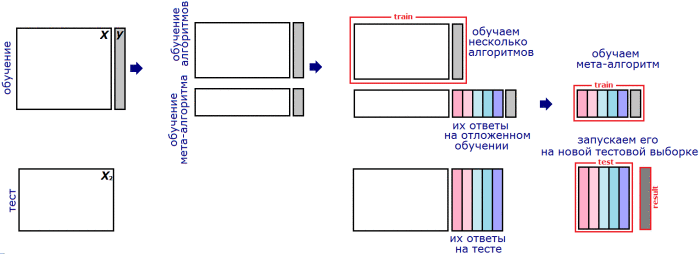Alexander Dyakonov (c)

## Stacking¶

### Weighted averaging¶

Consider regression with $K$ predictor models $f_{k}(x)$, $k=1,2,...K$.

(Alternatively we may consider $K$ discriminant functions in classification)

Weighted averaging combiner
$$f(x)=\sum_{k=1}^{K}w_{k}f_{k}(x)$$

Naive fitting $$\widehat{w}=\arg\min_{w}\sum_{i=1}^{N}\mathcal{L}(y_{i},\sum_{k=1}^{K}w_{k}f_{k}(x_{i}))$$

overfits. The mostly overfitted method will get the most weight.

## Linear stacking¶

• Let training set $\{(x_{i},y_{i}),i=1,2,...N\}$ be split into $M$ folds.
• Denote $fold(i)$ to be the fold, containing observation $i$
• Denote $f_{k}^{-fold(i)}$ be predictor $k$ trained on all folds, except $fold(i)$.

Definition</br>

Linear stacking is weighted averaging combiner, where weights are found using $$\widehat{w}=\arg\min_{w}\sum_{i=1}^{N}\mathcal{L}(y_{i},\sum_{k=1}^{K}w_{k}f_{k}^{-fold(i)}(x_{i}))$$

## General stacking¶

Definition

Generalized stacking is prediction $$f(x)=A_{\theta}\left(f_{1}(x),f_{2}(x),\ldots f_{K}(x)\right),$$

where $A$ is some general form predictor and $\theta$ is a vector of parameters, estimated by $$\widehat{\theta}=\arg\min_{\theta}\sum_{i=1}^{N}\mathcal{L}\left(y_{i},\,A_{\theta}\left(f_{1}^{-fold(i)}(x),f_{2}^{-fold(i)}(x),\ldots f_{K}^{-fold(i)}(x)\right)\right)$$

• Stacking is the most general approach
• It is a winning strategy in most ML competitions.
• $f_{i}(x)$ may be:
• class number (coded using one-hot encoding).
• vector of class probabilities
• any initial or generated feature

## General stacking¶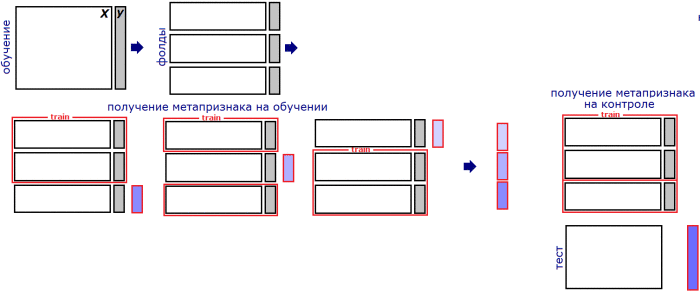Alexander Dyakonov (c)

• See this for additional details